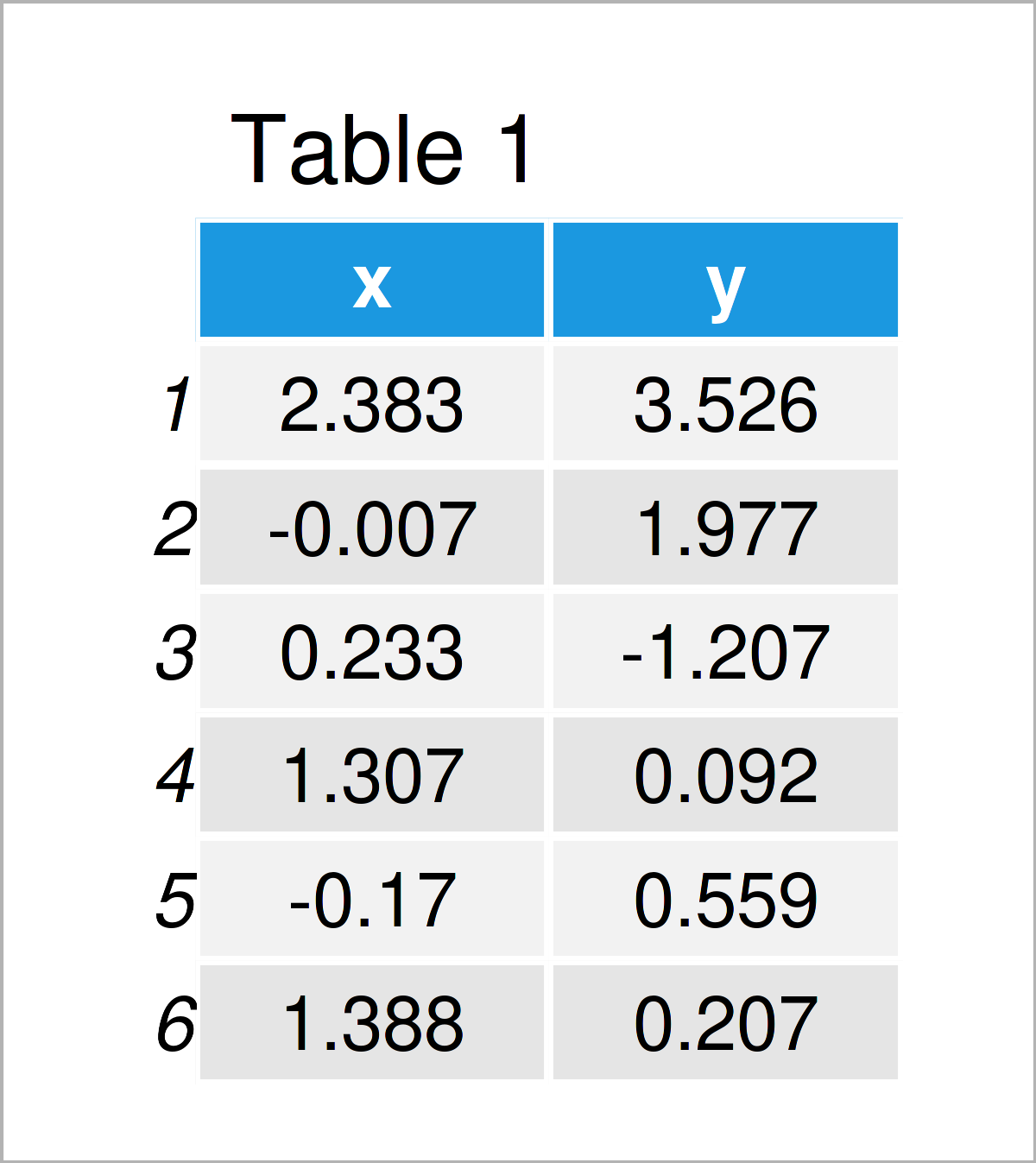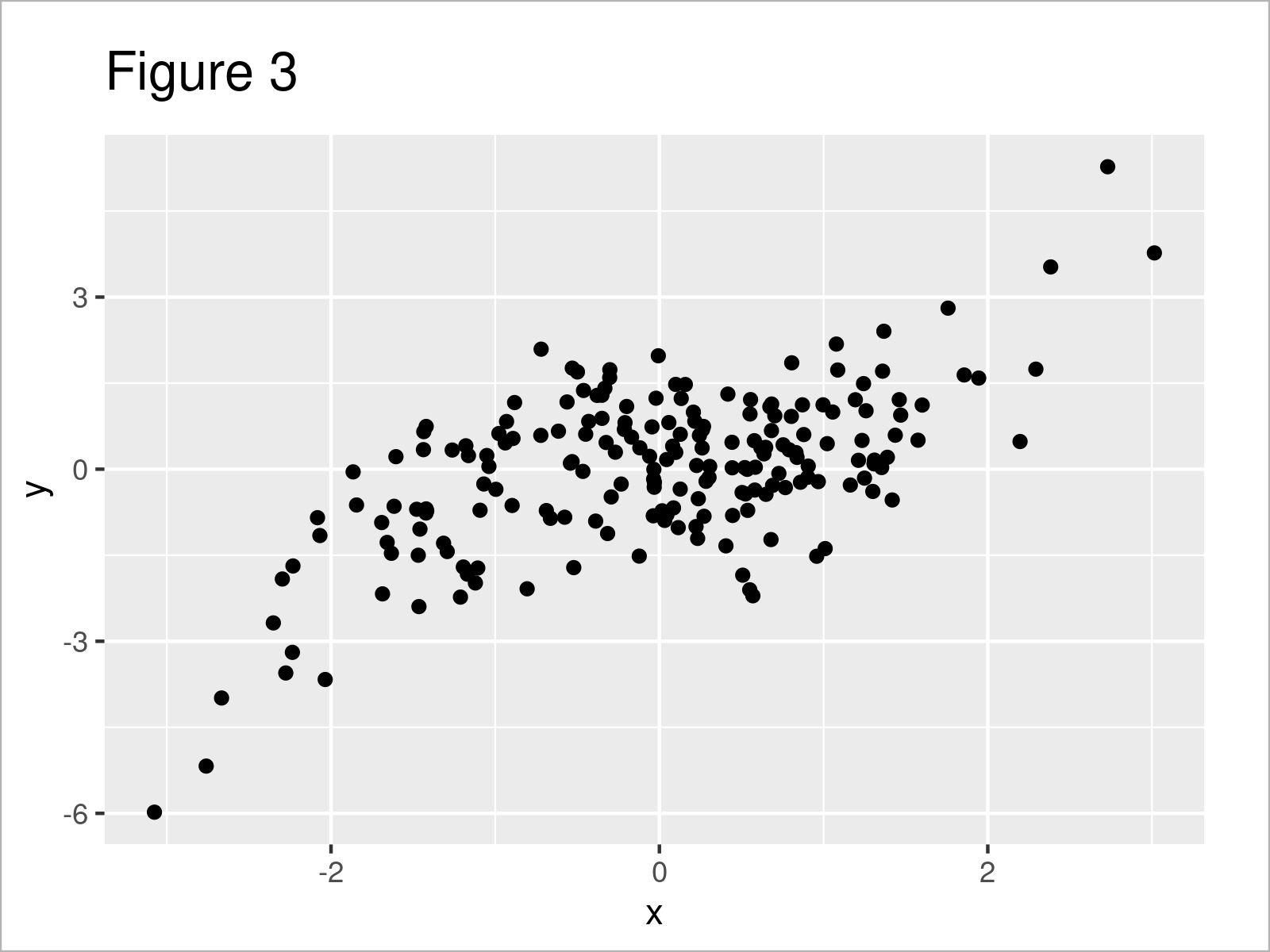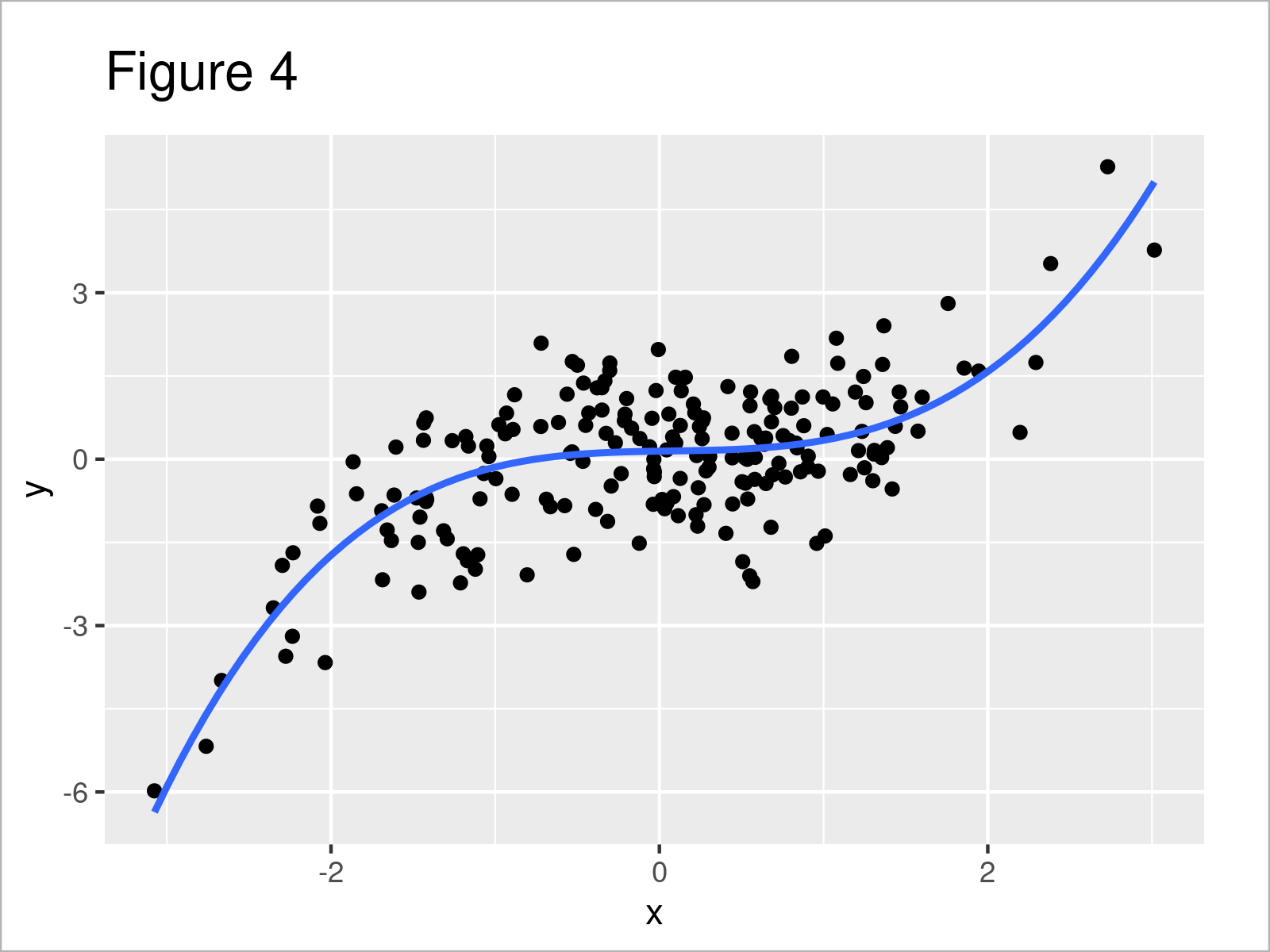# Add Polynomial Regression Line to Plot in R (2 Examples) | Base R & ggplot2

In this article, I’ll demonstrate how to draw a polynomial regression line in R programming.

The article consists of two examples for the addition of a polynomial regression line to a graph. To be more precise, the page will contain the following contents:

Let’s jump right to the examples!

## Creation of Example Data

The following data will be used as a basis for this R tutorial:

```set.seed(7355826)                   # Create example data frame
x <- rnorm(200)
y <- rnorm(200) + 0.2 * x^3
data <- data.frame(x, y)
head(data)                          # Print example data frame```Table 1 shows the head of our example data: it is also visualized that our data consists of two numerical columns.

Next, we can estimate a polynomial regression model of our data using the lm() function. We are using the variable x as predictor and the variable y as target variable.

```my_mod <- lm(y ~ poly(x, 4),        # Estimate polynomial regression model
data = data)
summary(my_mod)                     # Summary statistics of polynomial regression model
# Call:
# lm(formula = y ~ poly(x, 4), data = data)
#
# Residuals:
#      Min       1Q   Median       3Q      Max
# -2.39966 -0.59298  0.05659  0.71013  2.07603
#
# Coefficients:
#              Estimate Std. Error t value Pr(>|t|)
# (Intercept) -0.002771   0.067208  -0.041   0.9672
# poly(x, 4)1 11.898044   0.950459  12.518  < 2e-16 ***
# poly(x, 4)2 -2.125837   0.950459  -2.237   0.0264 *
# poly(x, 4)3  7.945027   0.950459   8.359 1.18e-14 ***
# poly(x, 4)4 -0.202329   0.950459  -0.213   0.8316
# ---
# Signif. codes:  0 ‘***’ 0.001 ‘**’ 0.01 ‘*’ 0.05 ‘.’ 0.1 ‘ ’ 1
#
# Residual standard error: 0.9505 on 195 degrees of freedom
# Multiple R-squared:  0.5429,	Adjusted R-squared:  0.5336
# F-statistic: 57.91 on 4 and 195 DF,  p-value: < 2.2e-16```

The previous output shows some descriptive statistics for our model. Let’s draw our data and the corresponding polynomial regression line!

## Example 1: Draw Polynomial Regression Curve to Base R Plot

The following R syntax shows how to create a scatterplot with a polynomial regression line using Base R.

Let’s first draw our data in a scatterplot without regression line:

`plot(y ~ x, data)                   # Draw Base R plot`In Figure 1 you can see that we have created a scatterplot showing our independent variable x and the corresponding dependent variable y.

Next, we can apply the lines function to add a polynomial regression curve on top of this plot:

```lines(sort(data\$x),                 # Draw polynomial regression curve
fitted(my_mod)[order(data\$x)],
col = "red",
type = "l")```After executing the previous syntax the scatterplot with polynomial regression line shown in Figure 2 has been plotted.

## Example 2: Draw Polynomial Regression Curve to ggplot2 Plot

Example 2 shows how to use the ggplot2 package to add a polynomial regression line to a graphic.

To be able to use the functions of the ggplot2 package, we first have to install and load ggplot2:

```install.packages("ggplot2")         # Install & load ggplot2 package
library("ggplot2")```

Next, we can visualize our data in a ggplot2 scatterplot without regression curve as shown below:

```ggp <- ggplot(data, aes(x, y)) +    # Create ggplot2 scatterplot
geom_point()
ggp                                 # Draw ggplot2 scatterplot```Figure 3 shows the output of the previous R code – A ggplot2 scatterplot.

In the next step, we can add a polynomial regression line to our ggplot2 plot using the stat_smooth function:

```ggp +                               # Add polynomial regression curve
stat_smooth(method = "lm",
formula = y ~ poly(x, 4),
se = FALSE)```After executing the previous R syntax the ggplot2 scatterplot with polynomial regression line shown in Figure 4 has been created.

If we want, we can also add confidence bands to our polynomial regression line.

For this, we have to set the se argument to be equal to TRUE (i.e. the default specification of the ggplot2 package):

```ggp +                               # Regression curve & confidence band
stat_smooth(method = "lm",
formula = y ~ poly(x, 4))```The output of the previous R programming code is shown in Figure 5 – A ggplot2 xyplot with polynomial regression line and standard errors for this regression line.

## Video & Further Resources

Would you like to learn more about the addition of a polynomial regression line to a graph? Then you may want to have a look at the following video on my YouTube channel. I illustrate the R syntax of this article in the video.

Please accept YouTube cookies to play this video. By accepting you will be accessing content from YouTube, a service provided by an external third party.If you accept this notice, your choice will be saved and the page will refresh.

In addition, you might read the related R tutorials on my website. Some posts are listed below.

In this R tutorial you have learned how to add a polynomial regression line to a plot. In case you have additional questions, let me know in the comments section.

Subscribe to the Statistics Globe Newsletter